Courses

# Test: Previous Year Questions: Classification Of Elements & Periodicity In Properties

## 24 Questions MCQ Test Chemistry for JEE | Test: Previous Year Questions: Classification Of Elements & Periodicity In Properties

Description
This mock test of Test: Previous Year Questions: Classification Of Elements & Periodicity In Properties for JEE helps you for every JEE entrance exam. This contains 24 Multiple Choice Questions for JEE Test: Previous Year Questions: Classification Of Elements & Periodicity In Properties (mcq) to study with solutions a complete question bank. The solved questions answers in this Test: Previous Year Questions: Classification Of Elements & Periodicity In Properties quiz give you a good mix of easy questions and tough questions. JEE students definitely take this Test: Previous Year Questions: Classification Of Elements & Periodicity In Properties exercise for a better result in the exam. You can find other Test: Previous Year Questions: Classification Of Elements & Periodicity In Properties extra questions, long questions & short questions for JEE on EduRev as well by searching above.
QUESTION: 1

### Which is the correct order of ionic sizes (At. No. : Ce = 58, Sn = 50, Yb = 70 and Lu = 71)                [AIEEE-2002]

Solution:

Generally as we move from left to right in a period, there is regular decrease in atomic radii and in a group as the atomic number increases the atomic radii also increases. Thus the atomic radius of Sn should be less than lanthanides. La > Sn. But due to lanthanide contraction, in case of lanthanides there is a continuous decrease in size with increase in atomic number. Hence the atomic radius follow the given trend:

Ce > Sn > Yb > Lu.

QUESTION: 2

### Ce3+, La3+, Pm3+ and Yb3+ have ionic radii in the increasing order as -                           [AIEEE-2002]

Solution:

Yb3+ < Pm3+< Ce3+<La3+

Due to the lanthanide contraction size of +3 ions decreases from La3+ to Lu3+

QUESTION: 3

### According to the Periodic Law of elements, the Variation in properties of elements is related to their ? [AIEEE-2003]

Solution:

According to modern periodic law, the properties of the elements are repeated after certain regular intervals when these elements are arranged in order of their increasing atomic numbers.

QUESTION: 4

The reduction in atomic size with increase in atomic number is a characteristic of elements of -                              [AIEEE-2003]

Solution:

With increase in atomic number i.e. in moving down a group, the number of the principal shell increases and therefore, the size of the atom increases. But in case of f ­block elements there is a steady decrease in atomic size with increase in atomic number due to lanthanide contraction. As we move through the lanthanide series, 4f electrons are being added one at each step.

The mutual shielding effect of f electrons is very little. This is due to the shape of the f ­orbitals. The nuclear charge, however increases by one at each step. Hence, the inward pull experienced by the 4f electrons increases. This causes a reduction in the size of the entire 4fn shell.

QUESTION: 5

Which one of the following groups represent a collection of isoelectronic species ?

(At. no. Cs = 55, Br = 35)                                                                                         [AIEEE-2003]

Solution:

Isoelectronic species are the neutral atoms, cations or anions of different elements which have the same number of electrons but different nuclear charge. N3^-, F^– and Na^+ each have 10 electr

QUESTION: 6

The atomic numbers of vanadium (V). Chromium (Cr), manganese (Mn) and iron (Fe) respectively 23, 24, 25 and 26. Which one of these may be expected to have the higher second ionization enthalpy ?

[AIEEE-2003]

Solution:

The electronic configurations are as follows;

V:(Ar)3d34s2

Cr:(Ar)3d54s1

Mn:(Ar)3d54s2

Fe:(Ar)3d64s2

The second ionization of Cr means removal of electron from the stable configuration of 3d5.

The highest second ionization enthalpy is Cr

Hence a is the correct answer.

QUESTION: 7

Which one of the following sets of ions represents the collection of isoelectronic species ?           [AIEEE-2004]

Solution:

Isoelectronic species are those which have same number of electrons.

K+ = 19 – 1 = 18 ; Ca2+ = 20 – 2 = 18

Sc3+ = 21 – 3 = 18 ; Cl = 17 + 1 = 18

Thus all these ions have 18 electrons in them.

QUESTION: 8

Which one of the following ions has the highest value of ionic radius ?                               [AIEEE-2004]

Solution:

This can be explained on the basis of z/e, where as z/e ratio increases, the size decreases and when z/e ratio decreases the size increases.

z is atomic number and e is number of electrons.

For Li+, z/e = 3/2 = 1.5

For F-, z/e = 9/10 = 0.9

For O2-, z/e = 8/10 = 0.8

For B3+, z/e = 5/2 = 2.5

The correct option is A.

QUESTION: 9

Among Al2O3, SiO2, P2O3 and SO2 the correct order of acid strength is :

[AIEEE-2004]

Solution:

While moving along a group from top to bottom acidic nature of oxides decreases and along a period left to right, acidic nature increases.
Al, Si, P, S

QUESTION: 10

The formation of the oxide ion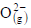requires first an exothermic and then an endothermic step as shown below:

O(g) + e- = O-(g)                 ΔH° = -142 kJ mol-1

O-(g) + e- = O2-(g)             ΔH° = 844 kJ mol-1

This is because of :

[AIEEE-2004]

Solution: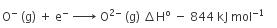This process is unfavourable in the gas phase because the resulting increase in electron-electron repulsion overweights the stability gained by achieving the noble gas configuration.

QUESTION: 11

In which of the following arrangements the order is NOT according to the property indicated against it?

[AIEEE-2005]

Solution:

Nitrogen has a stable configuration.

So, ionization enthalpy of nitrogen is greater than that of Oxygen, so the correct order is (B)B<C<N<O: Increasing first ionization energy

QUESTION: 12

Which of the following oxides is amphoteric in character ?                                                              [AIEEE-2005]

Solution: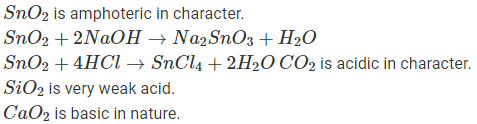QUESTION: 13

Pick out the isoelectronic structure from the following :                                                              [AIEEE-2005]

I. +CH3

II. H3O+

III. NH3

IV. CH3-

Solution: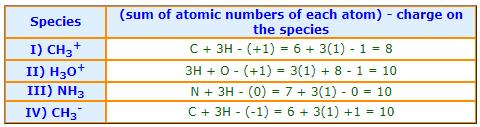H3O+, NH3 and CH3- are not only isoelectronic but are also iso-structural with pyramidal shape.

However CH3+ is trigonal planar with one empty orbital perpendicular to this plane.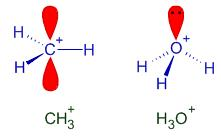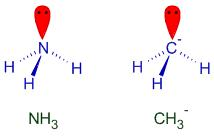QUESTION: 14

The lanthanide contraction is responsible for the fact that                                                              [AIEEE-2005]

Solution:

In each vertical column of transition elements, the elements of second and third transition series resemble each other more closely than the elements of first and second transition series on account of lanthanide contraction.

The pairs of elements such as Zr-­Hf, Mo-­W, Nb-­Ta, etc possess almost the same properties.

QUESTION: 15

Which of the following factors may be regarded as the main cause the lanthanide contraction ?

[AIEEE-2005]

Solution:

Lanthanide contraction is due to poor shielding of one of 4f electron by another in the sub-shell.

QUESTION: 16

The increasing order of the first ionization enthalpies of the elements B, P, S and F (lowest first) is–

[AIEEE-2006]

Solution:

In general as we move from left to right in a period, the ionisation enthalpy increases with increasing atomic number. The ionisation enthalpy decreases as we move down a group. P (1s2 2s2 2p6 3s2 3p3) has a stable half-filled electronic configuration than S (1s2 2s2 2p6 3s2 3p4). For this reason, ionization enthalpy of P is higher than S.

The correct option is D.

QUESTION: 17

Which one of the following sets of ions represents a collection of isoelectronic species ?             [AIEEE-2006]

Solution:

Each of K+, Cl-, Ca2+, Sc3+ has 18 electrons.

QUESTION: 18

lanthanoid contraction is caused due to -                              [AIEEE-2006]

Solution:

The Lanthanide Contraction is caused by a poor shielding effect of the 4f electrons. Gd because as atomic number increases, the atomic radius decreases. These electrons are do not shield good, causing a greater nuclear charge.

QUESTION: 19

Which one of the following constitutes a group of the isoelectronic species?                          [AIEEE-2008]

Solution:

Isoelectronic species are those species which have the same number of electrons. In the given set, option b has the same no. of electrons in all given molecules(14 e-).

QUESTION: 20

In which of the following arrangements, the sequence is not strictly according to the property written against it ?

[AIEEE-2009]

Solution:

On moving down the group, the basic tendency of hydrides of group 15 decreases.

QUESTION: 21

The correct sequence which shows decreasing order of the ionic radii of the elements is              [AIEEE-2010]

Solution:

O2-, F-, Na+, Mg2+,Al3+ are isoelectronic species (10 electrons each)
Cation having a positive charge is smaller in size than neutral atom whereas anion having a negative charge is larger in size than neutral atom.

QUESTION: 22

Which one of the following order represents the correct sequence of the increasing basic nature of the given oxides ?

[AIEEE-2011]

Solution:

While moving from left to right in periodic table basic character of oxide of elements will decrease.And while descending in the group basic character of corresponding oxides increases.QUESTION: 23

The outer electron configuration of Gd (Atomic No : 64 is :                   [AIEEE-2006]

Solution:

Gadolinium is a chemical element with symbol Gd and atomic number 64. It is a silvery-white, malleable and ductile rare-earth metal. It is found in nature only in combined form.

The outer electron configuration of Gd (Atomic No.: 64) is: Xe 4f5d6s2

QUESTION: 24

The increasing order of the ionic radii of the given isoelectronic species is:

Solution:

For isoelectronic species, as the z/e decreases, ionic radius increases
such as ionic radii ∝ 1/z.